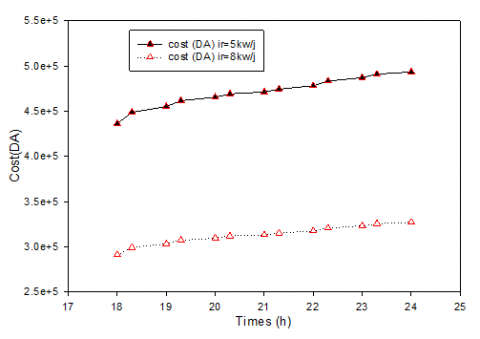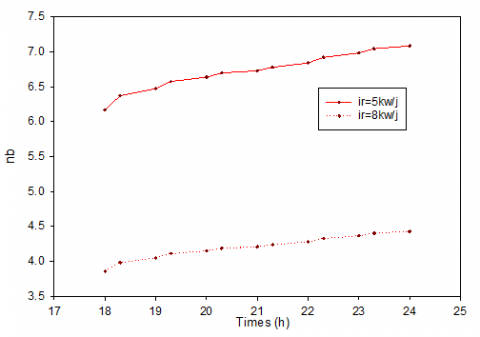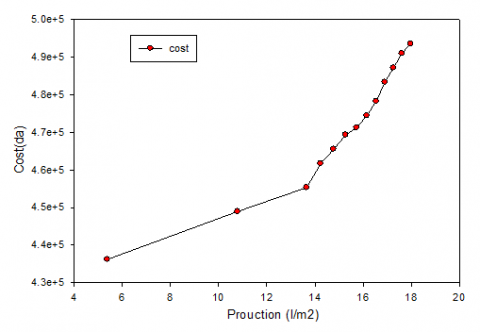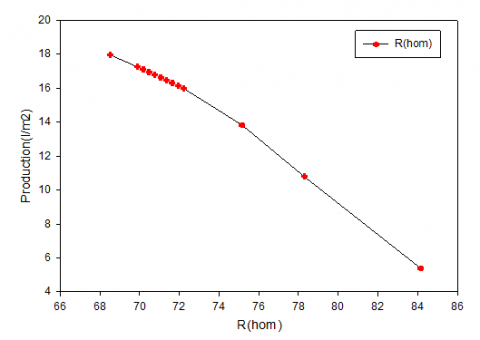# Effect of Various Physical Parameters on the Productivity of the Hybrid Distiller - In the Time of Distillation Extension at Night

Effect of Various Physical Parameters on the Productivity of the Hybrid Distiller - In the Time of Distillation Extension at Night

Benaissa Mandi* Younes Menni Ali J. Chamkha Giulio Lorenzini Noureddine Kaid Nacereddine Bibi-Triki Mohammed Bensafi Houari Ameur Djamel Sahel

Unit of Research on Materials and Renewable Energies, Department of Physics, Faculty of Sciences, Abou Bekr Belkaid University, B.P. 119, 13000, Tlemcen, Algeria

Mechanical Engineering Department, Prince Sultan Endowment for Energy and Environment, Prince Mohammad Bin Fahd University, Al-Khobar 31952, Saudi Arabia

Department of Engineering and Architecture, University of Parma, Parco Area delle Scienze, 181/A, Parma 43124, Italy

Laboratory of Energy in Arid Areas (ENERGARID) University of Bechar, B.P. 417, 08000, Bechar, Algeria

Department of Technology, University Center Salhi Ahmed of Naâma (Ctr Univ Naâma), B.P. 66, 45000, Naâma, Algeria

Laboratoire des Carburants Gazeux et Environnement, Faculté de Génie Mécanique, Université des Sciences et de la Technologie d’Oran, USTO-MB, BP1505, El-M’Naouer, Oran 31000, Algeria

Corresponding Author Email:
benaissamandi.2019@gmail.com
Page:
265-271
|
DOI:
https://doi.org/10.18280/ejee.210301
10 March 2019
|
Accepted:
3 May 2019
|
Published:
15 August 2019
| Citation

OPEN ACCESS

Abstract:

Through this recent simulation, we investigated the effect of different physical parameters on the productivity of the greenhouse still coupled with a cylindro-parabolic solar concentrator and an autonomous photovoltaic generator that prolongs the distillation time during the night. An attempt has been made to determine the various parameters that improve the productivity of the hybrid distiller during the day and at night. We identify new modifications and thermal and electrical storage mechanisms to achieve results, which illustrate the advantage and disadvantage of physical parameters to have a solar-based distillation that lasts 24 hours. This study will be a research opening to increase the productivity of solar distillers. Until we reached the water fill for a long time possible in all-weather cases by solar energy.

Keywords:

hybrid distillation, modeling, hybrid coupling with a cylindro parabolic concentrator, photovoltaic generator, thermal conversion, electrical conversion

1. Introduction

Solar distillation is an effective technique that reduces water scarcity in isolated sites as well as is a safe way against pollution and damage to human health. Effective modifications to solar distillers to produce enough water for society. Many processes are carried out to produce low cost water and reduced energy consumption as well as environmental concerns. Hosseini et al.  developed an active solar distillation system integrated with a solar parabolic through the concentrator and a vacuum-type heat exchanger. They experimentally evaluated its performance during the five days of October 2015. In their study, the effect of the environmental variables as well as the operational parameters on the performance of the whole solar distillation system was investigated regarding the total daily production rate. Ibrahim et al.  presented an exergoeconomic investigation for an evacuated solar distillation system that composed of still (evaporation chamber) and an air-cooled condenser (fresh water chamber). Their study covered exergetic, economic and exergoeconomic assessments of the solar distillation system. Jareanjit et al.  reported three scenarios for recycling the ethanol waste solution left behind in the tanks in each stage of distillation in a solar distillation system. Miao et al.  developed a membrane distillation device, consisting of a carbon nanotube membrane as solar absorption layer, a qualitative filter paper as water transmission pipeline and an aerogel blanket as thermal insulator to achieve efficient solar harvesting and heat localization for enhancing water evaporation. Moore et al.  developed a non-steady process model to simulate a sweeping gas membrane distillation system powered by solar thermal and photovoltaic power for the desalination of drinking water. Panthalookaran et al.  described experiments conducted to improve the efficiency of the dehumidifier attached to the novel solar distillation unit by optimizing the design parameters. Ranjan and Kaushik  presented a comprehensive survey and review of the efforts made in solar distillation systems and technologies with focus on the energy and exergy efficiency of the system. Saldivia et al.  conducted a numerical model for a multi-effect distillation (MED) plant driven by solar energy. Their model was based on mass, energy, and heat transfer equations applicable to the coupled MED and steam generation plants. Tan et al.  experimentally investigated the efficacy of MXene as a coating material on hydrophobic PVDF membranes to improve direct contact membrane distillation (DCMD) through the inherent photo-thermal and fouling mitigation functionalities. Tiwari et al.  performed an exergoeconomic and enviroeconomic analyses of partially covered photovoltaic thermal (PVT) flat plate collector (FPC) integrated solar distillation system known as PVT-FPC active solar distillation system. Their analysis was based on experimental studies for composite climatic condition of New Delhi. Tiwari and Sahota  highlighted an experimentally and theoretically detailed work done in recent past on passive and active solar stills. Yousef and Hassan  energetically, exergetically and economically investigated an experimental performance assessment of solar still system combined with PCM (phase change materials) storage unit. They used two techniques for further enhancing the still performance with PCM which are: embedded copper hollow pin fins (PF) inside the PCM and using mesh steel wool fibers (SWF) in the basin of the solar still with the PCM.

Zhu et al.  proposed and studied a novel light concentration and direct heating (LCDH) solar distillation device embedded underground in their analysis, to improve on the weakness of current solar distillers, especially complex structure, large floor space, inapplicability for large scale desalination. Other studies can be found in the literature as Li et al. , Wang et al. , Gopi et al. , Muraleedharan et al. , Parsa et al. , Miladi et al. 19], Hejazi et al. , Patel et al. , Afzal et al. , Al-Nimr et al. , An et al. , R. Bhardwaj et al. , Gupta et al. , Kiwan et al. , Ma et al. , Manchanda and Kumar , Reddy and Sharon , Reddy et al. , Tiwari et al. , Xu et al. , Zhang et al. , Zhou et al. , Sharan et al. , Shukla et al. , Al-Nimr et al. , Chafidz et al. , Chen et al. , Deshmukh and Thombre , Gakkhar et al. , Gil et al. , and Menni et al. [44-48].

In this numerical analysis, we simulate the effect of different physical parameters on the productivity of the greenhouse still coupled with a cylindro-parabolic solar concentrator and an autonomous photovoltaic generator that prolongs the distillation time during the night.

2. Hybrid Distillation Under Study

The considered physical model, reported in Figure 1, consists of a greenhouse still, its operation based on passive solar energy and a system for converting thermal energy via a cylindro-parabolic concentrator, an autonomous photovoltaic system or a wind generator. The latter feeds the system during the day and ensures the extension of operation in the night. The photovoltaic system has batteries for the purpose of storing electrical energy and reused during the absence of the radiant source, which is the sun. The duration of operation determines the sizing of the system. A long time increases the size of the photovoltaic system and increases the cost of installation. The high cost is a major disadvantage and pushes us to do some research to reduce the cost with a long distillation time during the night.

## 1.jpgFigure 1. Present hybrid distiller with a CCP and autonomous generator

3. Mathematical Foundation

3.1 Concentrator cylindro parabolic

Energy balance for the fluid

The energy balance for the coolant circulating in the absorber tube is:

$\frac{d}{d t}\left(\Delta Q_{f}(z, t)\right)=q_{i n l}(z, t)-q_{o u t}(z+\Delta z, t) \cdot \Delta z$ (1)

Energy balance for the absorber

The energy balance for the absorber is:

$\frac{\left.d\left(\Delta Q_{A}(z, t)\right)\right)}{d t}=\left(q_{a b s s o r b?}(t)\right)-q_{e n t r?}(z, t)-q_{u t i l e}(z, t) \cdot \Delta z$ (2)

Energy balance of the glass

The energy balance for the glass is:

$\rho_{v} C_{v} A_{v} \cdot \frac{d T_{v}(z, t)}{d t}=q_{\text {interne }}(z, t)-q_{\text {evene}}(z, t)$ (3)

$T_{v}(z, 0)=T_{v, \text { initial }}(z)=T_{a m b}$ (4)

3.2 Green house distiller

In the cover

Outside:

$\frac{M_{g}}{2} \cdot \frac{c p_{g}}{A_{g}} \cdot \frac{d T_{g e}}{d t}+q_{g-a}^{c}+q_{g-a}^{r}=\frac{\lambda_{g}}{e_{g}} \cdot\left(T_{g i}-T_{g e}\right)$ (5)

Inner face:

$\frac{M_{g}}{2} \cdot \frac{c p_{g}}{A_{g}} \cdot \frac{d T_{g i}}{d t}+\frac{\lambda_{g}}{e_{g}} \cdot\left(T_{g i}-T_{g e}\right)=q_{w-g}^{c}+q_{w-g}^{r}+q_{w-g}^{e v}+P_{g}$ (6)

In the brine

$M_{w} \cdot \frac{c p_{w}}{A_{w}} \cdot \frac{d T_{w}}{d t}+q_{w-g}^{c}+q_{w-g}^{r}+q_{w-g}^{e v}=p_{w}+q_{b-w}^{c}$ (7)

In the absorbent tray

$M_{b} \cdot \frac{c p_{b}}{A_{b}} \cdot \frac{d T_{b}}{d t}+q_{b-w}^{c}+q_{b-i s i}^{c d}=p b$ (8)

In the insulation

Inner face:

$\frac{M_{i s}}{2} \cdot \frac{c p_{i s}}{A_{i s}} \cdot \frac{d T_{i s i}}{d t}+\frac{\lambda_{i s}}{e_{i s}} \cdot\left(T_{i s i}-T_{i s e}\right)=q_{b-i s i}^{c d}$ (9)

Outside face:

$\frac{M_{i s}}{2} \cdot \frac{c p_{i s}}{A_{i s}} \cdot \frac{d T_{i s e}}{d t}+q_{i s-a}^{c}+q_{i s-a}^{r}=\frac{\lambda_{s s}}{e e_{i s}} \cdot\left(T_{s i s}-T_{i s e}\right)$ (10)

3.3 Hybrid distiller

$M_{w} \cdot \frac{c p_{w}}{A_{w}} \cdot \frac{d T_{w}}{d t}+q_{w-g}^{c}+q_{w-g}^{r}+q_{w-g}^{e v}=p_{w}+\operatorname{mcp}\left(T_{c c}-T_{w 0}\right)+\operatorname{mcp}\left(T_{e l}-T_{w 0}\right)+q_{b-w}^{c}$ (11)

3.4 Estimated consumption

$E_{A C}=\sum P_{(A C) i} t_{d i}$ （12）

$E_{D C}=\sum P_{(D C) i} t_{d i}$ (13)

$E_{T}=\frac{E_{D C}}{\eta_{B A T}}+\frac{E_{A C}}{\eta_{B A T} \cdot \eta_{I N V}}$ (14)

3.5 Dimensioning of the photovoltaic generator

$N_{T}=\frac{E_{T}}{P_{p} G_{m \beta} P_{G}}$ (15)

$N_{S}=\frac{V_{B a t}}{V_{m}}$ (16)

$N_{p}=\frac{N T}{N_{s}}$ (17)

3.6 Autonomy

$C_{n}(W h)=\frac{E_{T} N}{P_{d}}$ (18)

$C_{n}(A h)=\frac{C_{n}(W h)}{V_{b a t}}$ (19)

3.7 Sizing of the regulator

$I_{G}=I_{R} \cdot N_{R}$ (20)

$I_{R}=\frac{P_{p} \eta_{m}}{V_{m}}$ (21)

$I_{C}=\frac{P_{D C}}{V_{b a t m}}+\frac{P_{A C}}{220}$ (22)

$I_{R}=\max \left(I_{G}, I_{C}\right)$ (23)

3.8 Dimensioning of the converter

$P_{R i m v} \approx P_{A C}$ (24)

3.9 Dimensioning of the wiring

$P_{P C}=I^{2} \cdot R_{c}$ （25）

$R_{C}=\frac{\rho \cdot L}{S}$ (26)

3.10 Conversion of electrical energy to thermal energy

$Q=m \cdot C p \cdot \Delta T$ (27)

$P=V \cdot R=R \cdot I^{2}$ (28)

$Q=P . \Delta t$ (29)

$\Delta T=P . \Delta t / m . C p$ (30)

4. Results and Discusion

Figure 2 shows the variation of the cost of installation according to the electrical resistance, the increase of the resistance causes the increase of the cost of the autonomous system. Figures 3, and 4, 5 show the irradiation effect during the day on the number of solar panels and the number of batteries, which increase when Ir = 5 kw/d and decrease when Ir = 8 kw/d, so solar irradiation causes an inversely proportional effect with photovoltaic sizing.

## 2.pngFigure 2. Effect of electrical resistance on installation cost

## 3.pngFigure 3. Solar irradiation effect on the number of solar panels depending on the distillation time

Figure 6 shows the variation of the cost from 18h to 24h which increases with the increase of the production under the effect of the absence of the solar rays and the lowering of the outside temperature. Figures 7 and 8 Show the variation of the installation cost according to number of solar panels and number of storage batteries respectively. The increase of the two elements causes an increase in the cost of installation and the enlargement of the system.

## 4.pngFigure 4. Effect of irradiation on the cost of installation

## 5.pngFigure 5. Irradiation effect on the battery number

## 6.pngFigure 6. Variation of cost according to production

Figure 9 shows the variation of the production according to the electrical resistance. The increase in resistance keeps production at average values according to the condition of autonomy. The resistance is increased to recover the lowering of the temperature, which decreases under the influence of external parameters, such as the outside temperature and the absence of solar rays.

## 7.pngFigure 7. Variation of cost according to the number of panels

## 8.pngFigure 8. Variation of cost with battery number.

## 9.pngFigure 9. Effect of resistance on production from 18h to 24h

Figure 10 shows the variation of the water temperature according to the electrical resistance. The increase in resistance causes an increase in the temperature of the water and the opposite because of the thermal electrical conversion necessary for the system.

Figure 11 shows the variation of the thermal power as a function of the temperature of the water. The increase in temperature increases the thermal power and the opposite because of the thermal characteristics of the water.

## 10.pngFigure 10. Variation of the water temperature according to the electrical resistance

## 11.pngFigure 11. Variation of thermal power in function the temperature of the water

5. Conclusion

Research shows that there are several possibilities for increasing the productivity of the solar distiller and reducing the effect of factors that reduce production. Production by improving the storage of thermal energy to electricity with a system of cogeneration of solar photovoltaic and thermal energy produced by solar concentrators. It exceeds the production of other distillers. However, this study allows us to propose new designs that can offer better performance, a large amount of production and an extension of the distillation time of a hybrid distiller.

The increase in resistance causes the cost of the autonomous system to increase.

Solar irradiation causes an effect inversely proportional with photovoltaic sizing.

The cost of 18h to 24h which increases with the increase of the production under the effect of the absence of the solar rays and the lowering of the outside temperature.

The increase in the number of solar panels and the number of storage batteries, causes an increase in the cost of installation and the enlargement of the system.

The increase of the electrical resistance keeps the production at average values according to the condition of autonomy. The resistance increases to recover the lowering of the temperature which decreases under the influence of the external parameters like the outside temperature and the absence of the solar rays. The temperature increases the thermal power and the opposite because of the thermal characteristics of the water (proportional connection).

References

 Hosseini, A., Banakar, A., Gorjian, S. (2018). Development and performance evaluation of an active solar distillation system integrated with a vacuum-type heat exchanger. Desalination, 435: 45-59. https://doi.org/10.1016/j.desal.2017.12.031

 Ibrahim, A.G.M., Rashad, A.M., Dincer, I. (2017). Exergoeconomic analysis for cost optimization of a solar distillation system. Solar Energy, 151: 22-32. https://doi.org/10.1016/j.solener.2017.05.020

 Jareanjit, J., Siangsukone, P., Wongwailikhit, K., Tiansuwan, J. (2015). Management of ethanol waste from the solar distillation process: Experimental and theoretical studies. Energy Conversion and Management, 89: 330-338. https://doi.org/10.1016/j.enconman.2014.10.007

 Miao, E.D., Ye, M.Q., Guo, C.L., Liang, L., Liu, Q., Rao, Z.H. (2019). Enhanced solar steam generation using carbon nanotube membrane distillation device with heat localization. Applied Thermal Engineering, 149: 1255-1264. https://doi.org/10.1016/j.applthermaleng.2018.12.123

 Moore, S.E., Mirchandani, S.D., Karanikola, V., Nenoff, T.M., Arnold, R.G., Sáeza, A.E. (2018). Process modeling for economic optimization of a solar driven sweeping gas membrane distillation desalination system. Desalination, 437: 108-120. https://doi.org/10.1016/j.desal.2018.03.005

 Panthalookaran, V., Chettiyadan, D., Vadacherry, J., Kudakasseril, K., Parekkadan, V. (2016). Design of a helical coil dehumidifier for a novel gravity-driven solar distillation unit. Energy Procedia, 91: 294-302. https://doi.org/10.1016/j.egypro.2016.06.221

 Ranjan, K.R., Kaushik, S.C. (2013). Energy, exergy and thermo-economic analysis of solar distillation systems: A review. Renewable and Sustainable Energy Reviews, 27: 709-723. https://doi.org/10.1016/j.rser.2013.07.025

 Saldivia, D., Rosales, C., Barraza, R., Cornejo, L. (2019). Computational analysis for a multi-effect distillation (MED) plant driven by solar energy in Chile. Renewable Energy, 132: 206-220. https://doi.org/10.1016/j.renene.2018.07.139

 Tan, Y.Z., Wang, H., Han, L., Tanis-Kanbur, M.B., Pranav, M.V., Chew, J.W. (2018). Photothermal-enhanced and fouling-resistant membrane for solar-assisted membrane distillation. Journal of Membrane Science, 565: 254-265. https://doi.org/10.1016/j.memsci.2018.08.032

 Tiwari, G.N., Yadav, J.K., Singh, D.B., Al-Helal, I.M., Abdel-Ghany, A.M. (2015). Exergoeconomic and enviroeconomic analyses of partially covered photovoltaic flat plate collector active solar distillation system. Desalination, 367: 186-196. https://doi.org/10.1016/j.desal.2015.04.010

 Tiwari, G.N., Sahota, L. (2017). Review on the energy and economic efficiencies of passive and active solar distillation systems. Desalination, 401: 151-179. https://doi.org/10.1016/j.desal.2016.08.023

 Yousef, M.S., Hassan, H. (2019). Energetic and exergetic performance assessment of the inclusion of phase change materials (PCM) in a solar distillation system. Energy Conversion and Management, 179: 349-361. https://doi.org/10.1016/j.enconman.2018.10.078

 Zhu, Z., Zheng, H., Wang, Q., Chen, M., Li, Z., Zhang, B. (2018). The study of a novel light concentration and direct heating solar distillation device embedded underground. Desalination, 447: 102-119. https://doi.org/10.1016/j.desal.2018.08.021

 Li, Q., Beier, L.J., Tan, J., Brown, C., Lian, B., Zhong, W., Wang, Y., Ji, C., Dai, P., Li, T., Clech, P.L., Tyagi, H., Liu, X., Leslie, G., Taylor, R.A. (2019). An integrated, solar-driven membrane distillation system for water purification and energy generation. Applied Energy, 237: 534-548. https://doi.org/10.1016/j.apenergy.2018.12.069

 Wang, Q., Liang, S., Zhu, Z., Wu, G., Su, Y., Zheng, H. (2019). Performance of seawater-filling type planting system based on solar distillation process: Numerical and experimental investigation. Applied Energy, 250: 1225-1234. https://doi.org/10.1016/j.apenergy.2019.05.048

 Gopi, G., Arthanareeswaran, G., Ismail, A.F. (2019). Perspective of renewable desalination by using membrane distillation. Chemical Engineering Research and Design, 144: 520-537. https://doi.org/10.1016/j.cherd.2019.02.036

 Muraleedharan, M., Singh, H., Udayakumar, M., Suresh, S. (2019). Modified active solar distillation system employing directly absorbing Therminol 55-Al2O3 nano heat transfer fluid and Fresnel lens concentrator. Desalination, 457: 32-38. https://doi.org/10.1016/j.desal.2019.01.024

 Parsa, S.M., Javadi D.Y., Rahbar, A., Majidniya, M., Aberoumand, S., Amidpour, Y., Amidpour, M. (2019). Experimental assessment on passive solar distillation system on Mount Tochal at the height of 3964 m: Study at high altitude. Desalination, 466: 77-88. https://doi.org/10.1016/j.desal.2019.05.010

 Miladi, R., Frikha, N., Kheiri, A., Gabsi, S. (2019). Energetic performance analysis of seawater desalination with a solar membrane distillation. Energy Conversion and Management, 185: 143-154. https://doi.org/10.1016/j.enconman.2019.02.011

 Hejazi, M.A.A., Bamaga, O.A., Al-Beirutty, M.H., Gzara, L., Abulkhair, H. (2019). Effect of intermittent operation on performance of a solar-powered membrane distillation system. Separation and Purification Technology, 220: 300-308. https://doi.org/10.1016/j.seppur.2019.03.055

 Patel, J., Markam, B.K., Maiti, S. (2019). Potable water by solar thermal distillation in solar salt works and performance enhancement by integrating with evacuated tubes. Solar Energy, 188: 561-572. https://doi.org/10.1016/j.solener.2019.06.026

 Afzal, A., Munir, A., Ghafoor, A., Alvarado, J.L. (2017). Development of hybrid solar distillation system for essential oil extraction. Renewable Energy, 113: 22-29. https://doi.org/10.1016/j.renene.2017.05.027

 Al-Nimr, M.A., Qananba, K.S. (2018). A solar hybrid system for power generation and water distillation. Solar Energy, 171: 92-105. https://doi.org/10.1016/j.solener.2018.06.019

 An, W., Chen, L., Liu, T., Qin, Y. (2018). Enhanced solar distillation by nanofluid-based spectral splitting PV/T technique: Preliminary experiment. Solar Energy, 176: 146-156. https://doi.org/10.1016/j.solener.2018.10.029

 Bhardwaj, R., ten Kortenaar, M.V., Mudde, R.F. (2013). Influence of condensation surface on solar distillation. Desalination, 326: 37-45. https://doi.org/10.1016/j.desal.2013.07.006

 Gupta, V.S., Singh, D.B., Mishra, R.K., Sharma, S.K., Tiwarid, G.N. (2018). Development of characteristic equations for PVT-CPC active solar distillation system. Desalination, 445: 266-279. https://doi.org/10.1016/j.desal.2018.08.009

 Kiwan, S., Al-Nimr, M., Abdel Salam, Q.I. (2018). Solar chimney power-water distillation plant (SCPWDP). Desalination, 445: 105-114. https://doi.org/10.1016/j.desal.2018.08.006

 Ma, S., Chiu, C.P., Zhu, Y., Tang, C.Y., Long, H., Qarony, W., Zhao, X., Zhang, X., Lo, W.H., Tsang, Y.H. (2017). Recycled waste black polyurethane sponges for solar vapor generation and distillation. Applied Energy, 206: 63-69. https://doi.org/10.1016/j.apenergy.2017.08.169

 Manchanda, H., Kumar, M. (2017). Performance analysis of single basin solar distillation cum drying unit with parabolic reflector. Desalination, 416: 1-9. https://doi.org/10.1016/j.desal.2017.04.020

 Reddy, K.S., Sharon, H. (2017). Energy-environment-economic investigations on evacuated active multiple stage series flow solar distillation unit for potable water production. Energy Conversion and Management, 151: 259-285. https://doi.org/10.1016/j.enconman.2017.08.064

 Reddy, K.S., Sharon, H., Krithika, D., Philip, L. (2018). Performance, water quality and enviro-economic investigations on solar distillation treatment of reverse osmosis reject and sewage water. Solar Energy, 173: 160-172. https://doi.org/10.1016/j.solener.2018.07.033

 Tiwari, A.K., Somwanshi, A. (2018). Techno-economic analysis of mini solar distillation plants integrated with reservoir of garden fountain for hot and dry climate of Jodhpur (India). Solar Energy, 160: 216-224. https://doi.org/10.1016/j.solener.2017.11.078

  Xu, Y., Ma, J., Liu, D., Xu, H., Cui, F., Wang, W. (2019).  Origami system for efficient solar driven distillation in emergency water supply. Chemical Engineering Journal, 356: 869-876. https://doi.org/10.1016/j.cej.2018.09.070

 Zhang, Y., Liu, L., Li, K., Hou, D., Wang, J. (2018). Enhancement of energy utilization using nanofluid in solar powered membrane distillation. Chemosphere, 112: 554-562. https://doi.org/10.1016/j.chemosphere.2018.08.114

 Zhou, J., Zhang, X., Sun, B., Su, W. (2018). Performance analysis of solar vacuum membrane distillation regeneration. Applied Thermal Engineering, 144: 571-582. https://doi.org/10.1016/j.applthermaleng.2018.08.052

 Sharan, P., Neises, T., McTigue, J.D., Turchi, C. (2019). Cogeneration using multi-effect distillation and a solar-powered supercritical carbon dioxide Brayton cycle. Desalination, 459: 20-33. https://doi.org/10.1016/j.desal.2019.02.007

 Shukla, D.L., Modi, K.V. (2019). Hybrid solar still-liquid desiccant regenerator and water distillation system. Solar Energy, 182: 117-133. https://doi.org/10.1016/j.solener.2019.02.043

 Al-Nimr, M.A., Kiwan, S.M., Talafha, S. (2016). Hybrid solar-wind water distillation system. Desalination, 395: 33-40. https://doi.org/10.1016/j.desal.2016.05.018

 Chafidz, A., Kerme, E.D., Wazeer, I., Khalid, Y., Ajbar, A., Al-Zahrani, S.M. (2016). Design and fabrication of a portable and hybrid solar-powered membrane distillation system. Journal of Cleaner Production, 133: 631-647. https://doi.org/10.1016/j.jclepro.2016.05.127

 Chen, W., Zou, C., Li, X., Li, L. (2017). Experimental investigation of SiC nanofluids for solar distillation system: Stability, optical properties and thermal conductivity with saline water based fluid. International Journal of Heat and Mass Transfer, 107: 264-270. https://doi.org/10.1016/j.ijheatmasstransfer.2016.11.048

 Deshmukh, H.S., Thombre, S.B. (2017). Solar distillation with single basin solar still using sensible heat storage materials. Desalination, 410: 91-98. https://doi.org/10.1016/j.desal.2017.01.030

 Gakkhar, N., Soni, M.S., Jakhar, S. (2016). Second law thermodynamic study of solar assisted distillation system: A review. Renewable and Sustainable Energy Reviews, 56: 519-535. https://doi.org/10.1016/j.rser.2015.11.076

 Gil, J.D., Roca, L., Zaragoza, G., Berenguel, M. (2018). A feedback control system with reference governor for a solar membrane distillation pilot facility. Renewable Energy, 120: 536-549. https://doi.org/10.1016/j.renene.2017.12.107

 Menni, Y., Azzi, A., Chamkha, A. (2019). Modeling and analysis of solar air channels with attachments of different shapes. International Journal of Numerical Methods for Heat & Fluid Flow, 29(5): 1815-1845. https://doi.org/10.1108/HFF-08-2018-0435

 Menni, Y., Azzi, A., Didi, F., Harmand, S. (2018). Computational fluid dynamical analysis of new obstacle design and its impact on the heat transfer enhancement in a specific type of air flow geometry. Computational Thermal Sciences 10(5): 421-447. https://doi.org/10.1615/ComputThermalScien.2018024416

 Menni, Y., Azzi, A., Chamkha, A. (2019). Enhancement of convective heat transfer in smooth air channels with wall-mounted obstacles in the flow path. Journal of Thermal Analysis and Calorimetry, 135(4): 1951-1976. https://doi.org/10.1007/s10973-018-7268-x

 Menni, Y., Chamkha, A.J., Azzi, A., Zidani, C., Benyoucef, B. (2019). Study of air flow around flat and arc-shaped baffles in shell-and-tube heat exchangers. Mathematical Modelling of Engineering Problems, 6(1): 77-84. https://doi.org/10.18280/mmep.060110

 Menni, Y., Chamkha, A., Lorenzini, G., Benyoucef, B. (2019). Computational fluid dynamics based numerical simulation of thermal and thermo-hydraulic performance of a solar air heater channel having various ribs on absorber plates. Mathematical Modelling of Engineering Problems, 6(2): 170-174. https://doi.org/10.18280/mmep.06020